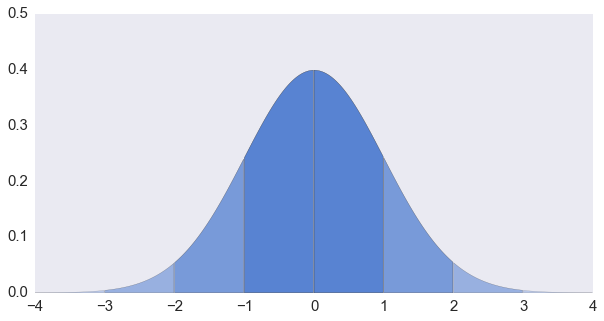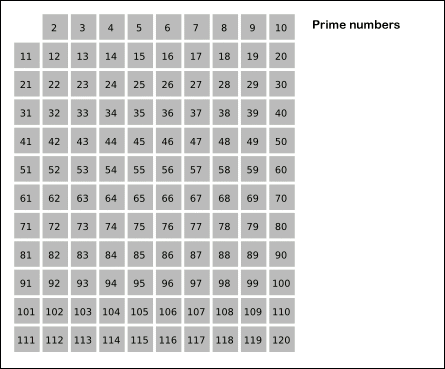# 正态分布随机数的生成 (2)

## 接受—拒绝法# 正态分布随机数的生成 (1)

$$f(x \; | \; \mu, \sigma) = \frac{1}{\sigma\sqrt{2\pi} } \; e^{ -\frac{(x-\mu)^2}{2\sigma^2} }$$# 蓄水池抽样浅说 (3)

#### 加个权吧

$$P_i = \frac{w_i}{w_1 + w_2 + \cdots + w_n}$$

$$P_n(1) = \frac{w_n}{w_1 + w_2 + \cdots + w_n}$$

$$P_{n-1}(2) = \frac{w_{n-1}}{w_1 + w_2 + \cdots + w_{n-1}}$$

$$P(S) = \prod_{i=1}^n \frac{w_i}{w_1+w_2+\cdots w_i}$$

# 蓄水池抽样浅说 (2)

#### 跳跳跳！—— Algorithm X

• 确定该跳过多少个元素 $S(k, n)$
• 跳过 $S(k, n)$ 个元素
• 从前 $k$ 个元素中随机产生一个要替换的元素，用下一个元素替换

# 蓄水池抽样浅说 (1)# 素数求和的动态规划方法# 0-1 背包问题详解 (4)# 0-1 背包问题详解 (3)

#### 哪能搞法——分支界限# 0-1 背包问题详解 (1)

#### 背包问题是啥